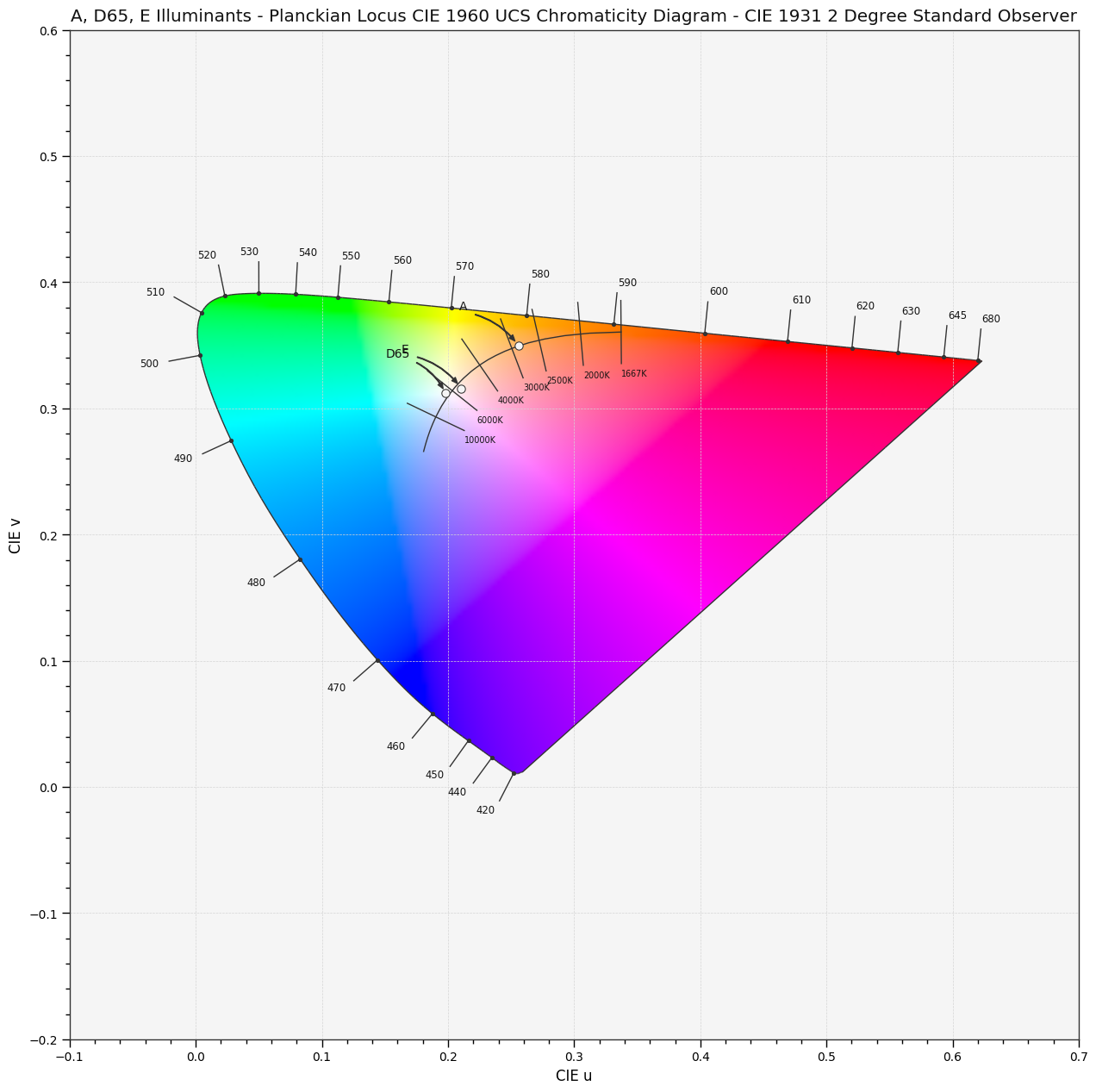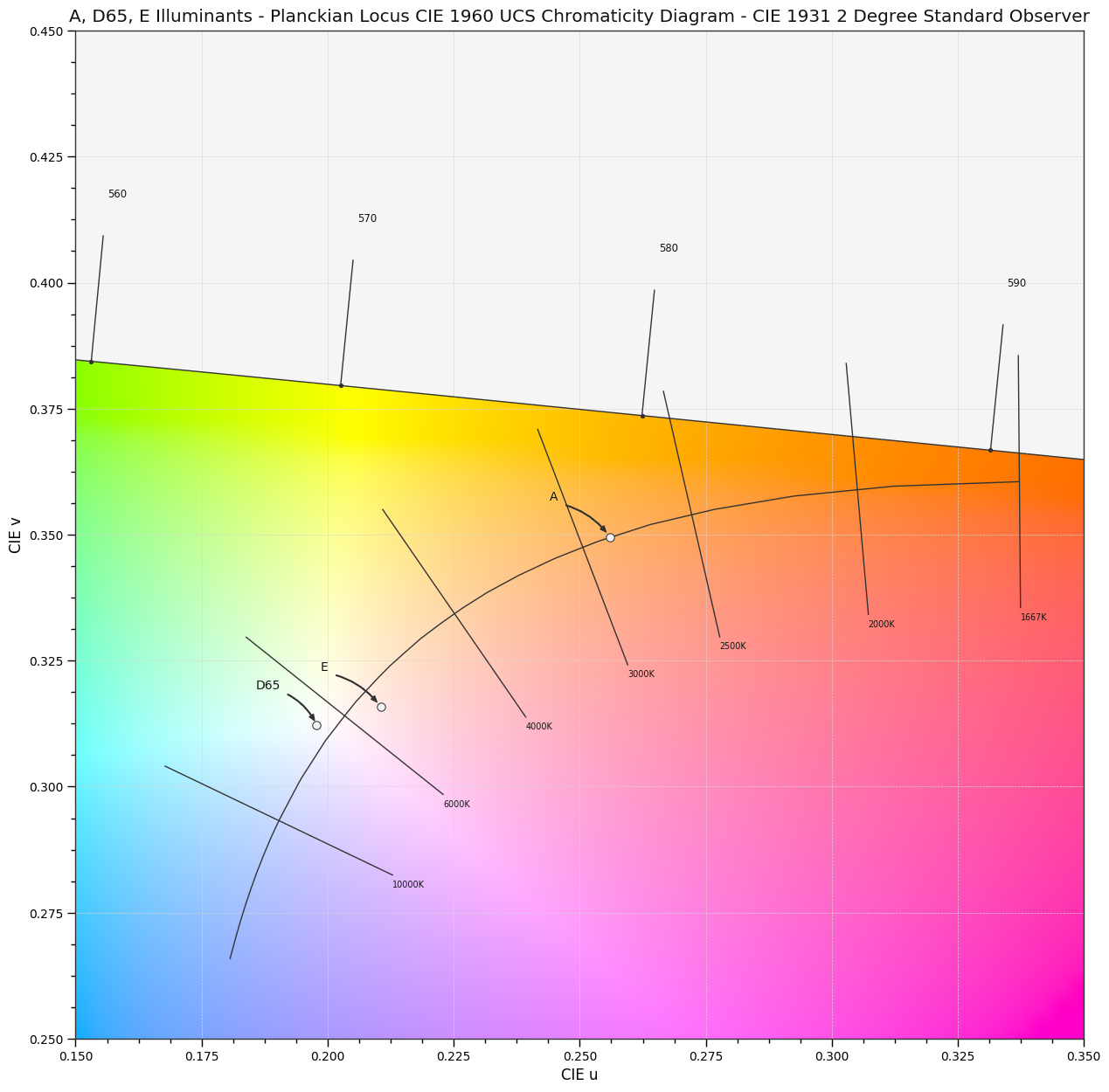Colour Temperature & Correlated Colour Temperature¶

The colour temperature noted $T_c$ is the temperature of a Planckian radiator whose radiation has the same chromaticity as that of a given stimulus. 

The correlated colour temperature noted $T_{cp}$ and shortened to $CCT$ is the temperature of the Planckian radiator having the chromaticity nearest the chromaticity associated with the given spectral distribution on a diagram where the (CIE 1931 2° Standard Observer based) $u^\prime, \cfrac{2}{3}v^\prime$ coordinates of the Planckian locus and the test stimulus are depicted. 

The CIE Standard Illuminant A, CIE Standard Illuminant D65 and CIE Illuminant E illuminants plotted in the CIE 1960 UCS Chromaticity Diagram:

In :
import colour

from colour.plotting import *
In :
colour_style();
In :
with colour.utilities.suppress_warnings(python_warnings=True):
plot_planckian_locus_in_chromaticity_diagram_CIE1960UCS(['A', 'D65', 'E']);In :
# Zooming into the *Planckian Locus*.
with colour.utilities.suppress_warnings(python_warnings=True):
plot_planckian_locus_in_chromaticity_diagram_CIE1960UCS(
['A', 'D65', 'E'], bounding_box=[0.15, 0.35, 0.25, 0.45]);The concept of correlated colour temperature should not be used if the chromaticity of the test source differs more than $\Delta C=5\cdot10^{-2}$ from the Planckian radiator with: 

$$\Delta C= \Biggl[ \Bigl(u_t^\prime-u_p^\prime\Bigr)^2+\cfrac{4}{9}\Bigl(v_t^\prime-v_p^\prime\Bigr)^2\Biggr]^{1/2}$$

where $u_t^\prime$, $u_p^\prime$ refer to the test source, $v_t^\prime$, $v_p^\prime$ to the Planckian radiator.

Colour implements various methods for correlated colour temperature computation $T_{cp}$ from chromaticity coordinates $xy$ or $uv$ and chomaticity coordinates $xy$ or $uv$ computation from correlated colour temperature:

• Robertson (1968) correlated colour temperature $T_{cp}$ and $D_{uv}$ computation method by interpolation between isotemperature lines.
• Ohno (2013) correlated colour temperature $T_{cp}$ and $D_{uv}$ computation method by direct approach and combined triangular and parabolic solutions.
• McCamy (1992) correlated colour temperature $T_{cp}$ cubic approximation computation method.
• Hernandez-Andres, Lee and Romero (1999) correlated colour temperature $T_{cp}$ cubic approximation computation method.
• Krystek (1985) chomaticity coordinates $uv$ polynomial approximation computation method.
• Kang et al. (2002) chomaticity coordinates $xy$ cubic approximation computation method.
• CIE Illuminant D Series chomaticity coordinates $xy$ computation method.

Robertson (1968) Method¶

Robertson (1968) method is based on $T_{cp}$ computation by linear interpolation between two adjacent members of a defined set of 31 isotemperature lines. 

In the CIE 1960 UCS chromaticity diagram the distance $d_i$ of the chromaticity point of given source ($u_s$, $v_s$) from each of the chromaticity point ($u_i$, $v_i$) through which the $i$th isotemperature line of slope $t_i$ passes is calculated as follows: 

$$\begin{equation} d_i=\cfrac{(v_s-v_i)-t_i(u_s-u_i)}{(1+t_i^2)^{1/2}} \end{equation}$$

The chromaticity point ($u_s$, $v_s$) is located between the adjacent isotemperature lines $j$ and $j + 1$ if $d_j/d_{j+1} < 0$

$$\begin{equation} T_c=\Biggl[\cfrac{1}{T_j}+\cfrac{\theta_1}{\theta_1+\theta_2}\biggl(\cfrac{1}{T_{j+1}}-\cfrac{1}{T_j}\biggr)\Biggr]^{-1} \end{equation}$$

where $\theta_1$ and $\theta_2$ are respectively the angles between the isotemperature lines $T_j$ and $T_{j+1}$ and the line joining ($u_s$, $v_s$) to their intersection. Since the isotemperature lines are narrow spaced $\theta_1$ and $\theta_2$ are small enough that one can set $\theta_1/\theta_2 = \sin\theta_1/\sin\theta_2$. The above equation can then be written:

$$\begin{equation} T_c=\Biggl[\cfrac{1}{T_j}+\cfrac{d_j}{d_j-d_{j+1}}\biggl(\cfrac{1}{T_{j+1}}-\cfrac{1}{T_j}\biggr)\Biggr]^{-1} \end{equation}$$

The colour.uv_to_CCT_Robertson1968 definition is used to calculate the correlated colour temperature $T_{cp}$ and distance $D_{uv}$ ($d_i$):

In :
colour.temperature.uv_to_CCT_Robertson1968((0.19783451566098664, 0.31221744678060825))
Out:
array([  6.50303994e+03,   3.25561654e-03])

colour.uv_to_CCT definition is implemented as a wrapper for various correlated colour temperature computation methods:

In :
colour.uv_to_CCT((0.19783451566098664, 0.31221744678060825), 'Robertson 1968')
Out:
array([  6.50303994e+03,   3.25561654e-03])

Note: 'robertson1968' is defined as a convenient alias for 'Robertson 1968':

In :
colour.uv_to_CCT((0.19783451566098664, 0.31221744678060825), 'robertson1968')
Out:
array([  6.50303994e+03,   3.25561654e-03])

Converting from correlated colour temperature $T_{cp}$ and distance $D_{uv}$ to chomaticity coordinates $uv$:

In :
colour.CCT_to_uv((6503.03994225557, 0.0032556165414977167), 'Robertson 1968')
Out:
array([ 0.19783447,  0.31221739])
In :
colour.CCT_to_uv((6503.03994225557, 0.0032556165414977167), 'robertson1968')
Out:
array([ 0.19783447,  0.31221739])

Ohno (2013) Method¶

Ohno (2013) presented new practical accurate methods to calculate the correlated colour temperature $T_{cp}$ and distance $D_{uv}$ with an error of 1 $K$ in $T_{cp}$ range from 1000 to 20,000 and $\pm$0.03 in $D_{uv}$. 

Triangular Solution¶

The correlated colour temperature is calculated by searching the closest point on the Planckian locus on the CIE 1960 UCS chromaticity diagram but without the complexity of Roberston (1968) method.

A table of coordinates ($U_i$, $V_i$) of Planckian locus (Planckian ($u$, $v$) table) in the estimated range of correlated colour temperature needed is generated and then the distance $d_i$ from the chromaticity coordinates ($U_x$, $V_x$) of a test light source is calculated.

The point $i = m$ is the point where $d_i$ is the smallest in the table ensuring that the correlated colour temperature to be obtained lies between $T_{m-1}$ and $T_{m+1}$.

The previous computation is repeated $n$ times through cascade expansion in order to reduce errors.

A triangle is then formed by the chromaticity point ($U_x$, $V_x$) of the test light soure and the chromaticity points on Planckian locus at $T_{m-1}$ and $T_{m+1}$. The blackbody temperature $T_x$ for the closest point to the line between $T_{m-1}$ and $T_{m+1}$ is calculated as follows: 

$$\begin{equation} T_x=T_{m-1}+(T_{m+1}-T_{m-1})\cdot\cfrac{x}{l} \end{equation}$$

with

\begin{equation} \begin{aligned} x&=\cfrac{d_{m-1}^2-d_{m+1}^2+l^2}{2l}\\ l&=\sqrt{(u_{m+1}-u_{m-1})^2+(v_{m+1}-v_{m-1})^2} \end{aligned} \end{equation}

$D_{uv}$ is then calculated as follows:

$$\begin{equation} D_{uv}=(d_{m-1}^2-x^2)^{1/2}\cdot sgn(v_x-v_{T_x}) \end{equation}$$

with

\begin{equation} \begin{aligned} v_{T_x}&=v_{m-1}+\{v_{m+1}-v_{m-1}\}\cdot x/l\\ SIGN(z)&=1\ for\ z \geq0\ and\ SIGN(z)=-1\ for\ z <0 \end{aligned} \end{equation}

Errors due to the non linearity of the correlated colour temperature scale on ($u$, $v$) coordinates are reduced by applying the following correction:

$$\begin{equation} T_{x,cor}=T_x\times 0.99991 \end{equation}$$

This correction is not needed for Planckian ($u$, $v$) table with steps of 0.25% or smaller.

Parabolic Solution¶

After finding $T_{m-1}$ and $T_{m+1}$ as described in the triangular solution method above, $d_{m−1}$, $d_m$, $d_{m+1}$ are fitted to a parabolic function. The polynomial is derived from $d_{m−1}$, $d_m$, $d_{m+1}$ and $T_{m−1}$, $T_m$, $T_{m+1}$ as: 

$$\begin{equation} d(T)=aT^2+bT+c \end{equation}$$

where

\begin{equation} \begin{aligned} a&\ =[T_{m-1}(d_{m+1}-d_m)+T_m(d_{m-1}-d_{m+1})+T_{m+1}(d_m-d_{m-1})]\cdot X^{-1}\\ b&\ =-[T_{m-1}^2(d_{m+1}-d_m)+T_m^2(d_{m-1}-d_{m+1})+T_{m+1}^2(d_m-d_{m-1})]\cdot X^{-1}\\ c&\ =-[d_{m-1}(T_{m+1}-T_m)\cdot T_m\cdot T_{m+1}+d_m(T_{m-1}-T_{m+1})\cdot T_{m-1}\cdot T_{m+1}+d_{m+1}(T_m-T_{m-1})\cdot T_{m-1}\cdot T_m]\cdot X^{-1} \end{aligned} \end{equation}

with

$$\begin{equation} X=(T_{m+1}-T_m)(T_{m-1}-T_{m+1})(T_m-T_{m-1}) \end{equation}$$

The correlated colour temperature $T=T_x$ is then obtained as follows:

$$\begin{equation} T_X=-\cfrac{b}{2a}\qquad\because d^\prime(T)=2aT_x+b=0 \end{equation}$$

The correction factor $T_{x,cor}$ for nonlinearity is applied as described in the triangular solution method.

$D_{uv}$ is then calculated as follows:

$$\begin{equation} D_{uv}=SIGN(v_x-v_{T_x})\cdot(aT_{x,cor}^2+bT_{x,cor}+c) \end{equation}$$

with

$$\begin{equation} SIGN(z)=1\ for\ z \geq0\ and\ SIGN(z)=-1\ for\ z <0 \end{equation}$$

Combined Solution¶

The parabolic solution works accurately except in on or near the Planckian locus. Taking triangular solution results for $|D_{uv}| < 0.002$ and the parabolic solution results for other regions solves that problem. 

The colour.uv_to_CCT_Ohno2013 definition is used to calculate the correlated colour temperature $T_{cp}$ and distance $D_{uv}$ ($d_i$):

In :
colour.temperature.uv_to_CCT_Ohno2013((0.19783451566098664, 0.31221744678060825))
Out:
array([  6.50364955e+03,   3.20563638e-03])

Precision can be changed by passing a value to the iterations argument:

In :
for i in range(10):
print(colour.temperature.uv_to_CCT_Ohno2013(
(0.19783451566098664, 0.31221744678060825), iterations=i + 1))
[  1.68848187e+04   2.66789445e-03]
[  6.79456522e+03   3.46904097e-03]
[  6.55383806e+03   3.48674101e-03]
[  6.50607833e+03   3.20681676e-03]
[  6.50374738e+03   3.20565144e-03]
[  6.50364955e+03   3.20563638e-03]
[  6.50364731e+03   3.20563610e-03]
[  6.50364722e+03   3.20563604e-03]
[  6.50364721e+03   3.20563264e-03]
[  6.50364721e+03   3.20561976e-03]

Using the colour.uv_to_CCT wrapper definition:

In :
colour.uv_to_CCT((0.19783451566098664, 0.31221744678060825), 'Ohno 2013')
Out:
array([  6.50364955e+03,   3.20563638e-03])

Note: 'ohno2013' is defined as a convenient alias for 'Ohno 2013':

In :
colour.uv_to_CCT((0.19783451566098664, 0.31221744678060825), 'ohno2013')
Out:
array([  6.50364955e+03,   3.20563638e-03])

Converting from correlated colour temperature $T_{cp}$ and distance $D_{uv}$ to chomaticity coordinates $uv$:

In :
colour.CCT_to_uv((6503.03994225557, 0.0032556165414977167), 'Ohno 2013')
Out:
array([ 0.19779726,  0.31225121])
In :
colour.CCT_to_uv((6503.03994225557, 0.0032556165414977167), 'Ohno 2013')
Out:
array([ 0.19779726,  0.31225121])

McCamy (1992) Method¶

McCamy (1992) proposed an equation to compute correlated colour temperature $T_{cp}$ from CIE 1931 2° Standard Observer chromaticity coordinates $x$, $y$ by using a chromaticity epicenter ($x_e$, $y_e$) where the isotemperature lines in some of the correlated colour temperature range converge and the inverse slope of the line $n$ that connects it to $x$, $y$. 

The cubic approximation equation is defined as follows: 

$$\begin{equation} T_{cp}=-449n^3+3525n^2-6823.3n+5520.33 \end{equation}$$

where

$$\begin{equation} n=\cfrac{x-x_e}{y-ye}\\ x_e=0.3320\qquad y_e=0.1858 \end{equation}$$

The colour.xy_to_CCT_mccamy definition is used to calculate the correlated colour temperature $T_{cp}$ from CIE 1931 2° Standard Observer chromaticity coordinates $x$, $y$:

In :
colour.temperature.xy_to_CCT_McCamy1992((0.31271, 0.32902))
Out:
6504.3893830489724

The colour.xy_to_CCT definition is implemented as a wrapper for various correlated colour temperature computation methods from CIE 1931 2° Standard Observer chromaticity coordinates $x$, $y$:

In :
colour.xy_to_CCT((0.31271, 0.32902), 'McCamy 1992')
Out:
6504.3893830489724

Note: 'mccamy1992' is defined as a convenient alias for 'McCamy 1992':

In :
colour.xy_to_CCT((0.31271, 0.32902), 'mccamy1992')
Out:
6504.3893830489724

Hernandez-Andres, Lee and Romero (1999) Method¶

Hernandez-Andres, Lee and Romero (1999) extended McCamy (1992) work by using a second epicenter to extend the accuracy over a wider correlated colour temperature and chromaticity coordinates range ($3000$–$10^6K$). 

The new extended equation to calculate the correlated colour temperature $T_{cp}$ is defined as follows: 

$$\begin{equation} T_{cp}=A_0+A_1exp(-n/t_1)+A_2exp(-n/t_2)+A_3exp(-n/t_3) \end{equation}$$

where

$$\begin{equation} n=\cfrac{x-x_e}{y-ye}\\ \end{equation}$$

with

Constants $T_{cp}$ Range ($K$) $3000$-$50,000$ $T_{cp}$ Range ($K$) $50,000$-$8\times10^5$
$A_0$ $-949.86315$ $36284.48953$
$A_1$ $6253.80338$ $0.00228$
$t_1$ $0.92159$ $0.07861$
$A_2$ $28.70599$ $5.4535\times10^{-36}$
$t_2$ $0.20039$ $0.01543$
$A_3$ $0.00004$
$t_3$ $0.07125$
$x_e$ $0.3366$ $0.3356$
$y_e$ $0.1735$ $0.1691$

The colour.xy_to_CCT_hernandez definition is used to calculate the correlated colour temperature $T_{cp}$ from CIE 1931 2° Standard Observer chromaticity coordinates $x$, $y$:

In :
colour.temperature.xy_to_CCT_Hernandez1999((0.31271, 0.32902))
Out:
6500.0421533365825

Using the colour.xy_to_CCT wrapper definition:

In :
colour.xy_to_CCT((0.31271, 0.32902), 'Hernandez 1999')
Out:
6500.0421533365825

Note: 'hernandez1999' is defined as a convenient alias for 'Hernandez 1999':

In :
colour.xy_to_CCT((0.31271, 0.32902), 'hernandez1999')
Out:
6500.0421533365825

Krystek (1985) Method¶

Krystek (1985) proposed a polynomial approximation valid from $1000K$ to $15000K$. 

The CIE UCS colourspace chromaticity coordinates $u$, $v$ are given by the following equations: 

\begin{equation} \begin{aligned} u&\ =\cfrac{0.860117757 + 1.54118254 \times 10^{-4} T + 1.28641212 \times 10^{-7} T^2}{1 + 8.42420235 \times 10^{-4} T + 7.08145163 \times 10^{-7} T^2}\\ v&\ =\cfrac{0.317398726 + 4.22806245 \times 10^{-5} T + 4.20481691 \times 10^{-8} T^2}{1 - 2.89741816 \times 10^{-5} T + 1.61456053 \times 10^{-7} T^2} \end{aligned} \end{equation}

The colour.CCT_to_uv_Krystek1985 definition is used to calculate the CIE UCS colourspace chromaticity coordinates $u$, $v$ from correlated colour temperature $T_{cp}$:

In :
colour.temperature.CCT_to_uv_Krystek1985(6504.389383048972)
Out:
array([ 0.18376696,  0.30934437])

Using the colour.CCT_to_uv wrapper definition:

In :
colour.CCT_to_uv(6504.389383048972, 'Krystek 1985')
Out:
array([ 0.18376696,  0.30934437])

Kang et al. (2002) Method¶

Kang et al. (2002) proposed an advanced colour-temperature control system for HDTV applications in the range from $1667K$ to $25000K$. 

The CIE 1931 2° Standard Observer chromaticity coordinates $x$, $y$ are given by the following equations: 

\begin{equation} \begin{aligned} x&\ =\begin{cases}-0.2661239\cfrac{10^9}{T_{cp}^3}-0.2343589\cfrac{10^6}{T_{cp}^2}+0.8776956\cfrac{10^3}{T_{cp}}+0.179910 & for\ 1667K\leq T_{cp}\leq4000k\\ -3.0258469\cfrac{10^9}{T_{cp}^3}+2.1070379\cfrac{10^6}{T_{cp}^2}+0.2226347\cfrac{10^3}{T_{cp}}+0.24039 & for\ 4000K\leq T_{cp}\leq25000k\end{cases}\\ y&\ =\begin{cases}-1.1063814x^3-1.34811020x^2+2.18555832x-0.20219683 & for\ 1667K\leq T_{cp}\leq2222k\\ -0.9549476x^3-1.37418593x^2+2.09137015x-0.16748867 & for\ 2222K\leq T_{cp}\leq4000k\\ 3.0817580x^3-5.8733867x^2+3.75112997x-0.37001483 & for\ 4000K\leq T_{cp}\leq25000k\end{cases} \end{aligned} \end{equation}

The colour.CCT_to_xy_kang definition is used to calculate the CIE 1931 2° Standard Observer chromaticity coordinates $x$, $y$ from correlated colour temperature $T_{cp}$:

In :
colour.temperature.CCT_to_xy_Kang2002(6504.389383048972)
Out:
array([ 0.313426  ,  0.32359597])

The colour.CCT_to_xy definition is implemented as a wrapper for various CIE 1931 2° Standard Observer chromaticity coordinates $x$, $y$ computation from correlated colour temperature:

In :
colour.CCT_to_xy(6504.389383048972, 'Kang 2002')
Out:
array([ 0.313426  ,  0.32359597])

Note: 'kang2002' is defined as a convenient alias for 'Kang 2002':

In :
colour.CCT_to_xy(6504.389383048972, 'kang2002')
Out:
array([ 0.313426  ,  0.32359597])

CIE Illuminant D Series Method¶

Judd et al. (1964) defined the following equations to calculate the CIE 1931 2° Standard Observer chromaticity coordinates $x_D$, $y_D$ of a CIE Illuminant D Series: 

\begin{equation} \begin{aligned} x_D&\ =\begin{cases}-4,6070\cfrac{10^9}{T_{cp}^3}+2.9678\cfrac{10^6}{T_{cp}^2}+0.09911\cfrac{10^3}{T_{cp}}+0.244063 & for\ 4000K\leq T_{cp}\leq7000k\\ -2.0064\cfrac{10^9}{T_{cp}^3}+1.9018\cfrac{10^6}{T_{cp}^2}+0.24748\cfrac{10^3}{T_{cp}}+0.237040 & for\ 7000K\leq T_{cp}\leq25000k\end{cases}\\ y_D&\ =-3.000x_D^2+0.2.870x_D-0.275 \end{aligned} \end{equation}

The colour.CCT_to_xy_CIE_D definition is used to calculate the CIE 1931 2° Standard Observer chromaticity coordinates $x$, $y$ of a CIE Illuminant D Series from correlated colour temperature $T_{cp}$:

In :
colour.temperature.CCT_to_xy_CIE_D(6504.389383048972)
Out:
array([ 0.31270775,  0.32911283])

Using the colour.CCT_to_xy wrapper definition:

In :
colour.CCT_to_xy(6504.389383048972, 'CIE Illuminant D Series')
Out:
array([ 0.31270775,  0.32911283])

Note: 'daylight' is defined as a convenient alias for 'CIE Illuminant D Series':

In :
colour.CCT_to_xy(6504.389383048972, 'daylight')
Out:
array([ 0.31270775,  0.32911283])